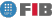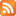# Computational Geometry (GEOC)

Credits Dept. Type Requirements
7.5 (6.0 ECTS) MAT
• Elective for DIE
• Elective for DCSFW
AL - Prerequisite for DIE , DCSYS
CAL - Prerequisite for DIE , DCSYS

## Instructors

 Person in charge: (-) Others: (-)

## General goals

We will present the most frequent geometric algorithms behind many computer science applications in fields such as computer graphics, visualization, shape reconstruction, computer vision, geographical information systems, robotics, etc.

We will give the appropriated tools for dealing with massive geometric data (algorithms and data structures) and will exploit the geometric properties of the problems posed, to find optimal solutions.

## Specific goals

### Knowledges

1. Learn the several kinds of problems in Computational Geometry, as well as their applications.
2. Learn the capacity of combining geometric tools with the appropriated data structures and algorithmic paradigms.
3. See in action several algorithmic paradigms and data structures useful in geometric problems.
4. Apply geometric results to real problems.

### Abilities

1. Ability to solve basic problems that appear in computational geometry.
2. Ability to implement the solutions proposed in the class, as well as those that can be found in the basic references of the course.
3. Ability to recognize the geometric problems behind the applications, and to propose adequate algorithmic tools to solve them.

### Competences

1. Ability to create and use real 2D and 3D geometric models.
2. Ability to analize problems: distinguish the geometric characteristics of the problem, properly describe the data (input) and precisely define the geometric information that we want to obtain (output).
3. Ability to tackle new problems by consciously using strategies that have proved useful in solving previous problems.

## Contents

Estimated time (hours):

 T P L Alt Ext. L Stu A. time Theory Problems Laboratory Other activities External Laboratory Study Additional time

1. Introduction to Computational Geometry
T      P      L      Alt    Ext. L Stu    A. time Total
2,0 0 0 0 0 1,0 0 3,0
The problems studied in Computational Geometry. Applications. Terminology. Basic Tools.

2. A basic tool
T      P      L      Alt    Ext. L Stu    A. time Total
2,0 1,0 3,0 0 3,0 1,0 0 10,0
Oriented area. Left/right. Intersection of two lines. Intersection of two segments. Oriented turn.

3. Basic geometric problems on polygons
T      P      L      Alt    Ext. L Stu    A. time Total
2,0 2,0 4,0 0 4,0 2,0 0 14,0
Line/polygon intersection, point location in a polygon, supporting lines to a polygon from a point, etc.

4. Convex hull
T      P      L      Alt    Ext. L Stu    A. time Total
4,0 2,0 0 0 0 3,0 0 9,0
Algorithms for the construction od the convex hull of 2D point sets. Convex hull in 3D.

5. Duality. Intersection of halfplanes. Linear programming.
T      P      L      Alt    Ext. L Stu    A. time Total
4,0 2,0 0 0 0 4,0 0 10,0
Geometric duality. The parabola duality. Intersection of halfplanes and convex hulls. Linear algorithm for LP problems.

6. Polygon triangulation
T      P      L      Alt    Ext. L Stu    A. time Total
4,0 2,0 0 0 0 3,0 0 9,0
Triangulation of monotone polygons, decomposition of a polygon into monotone polygons.

7. Proximity
T      P      L      Alt    Ext. L Stu    A. time Total
7,0 2,0 2,0 0 3,0 6,0 0 20,0
Voronoi Diagram

8. Triangulations of point sets
T      P      L      Alt    Ext. L Stu    A. time Total
5,0 2,0 5,0 0 10,0 4,0 0 26,0
Delaunay triangulation

9. Arrangements
T      P      L      Alt    Ext. L Stu    A. time Total
4,0 1,0 0 0 0 3,0 0 8,0
Arrengements of lines. Arrengements of segments.

10. Location
T      P      L      Alt    Ext. L Stu    A. time Total
2,0 0 0 0 0 1,0 0 3,0
Point location in planar decompositions.

11. Students presentations of other problems
T      P      L      Alt    Ext. L Stu    A. time Total
6,0 0 0 0 10,0 12,0 0 28,0
Extensions of the course contents.

 Total per kind T P L Alt Ext. L Stu A. time Total 42,0 14,0 14,0 0 30,0 40,0 0 140,0 Avaluation additional hours 7,0 Total work hours for student 147,0

## Docent Methodolgy

Theory classes will set out the contents of the course, oriented to the resolution of examples and applications.

Exercise classes will be centered in the resolution of problems by the students. Students will be assigned problems and will have enough time to think about them in advance, so that they will be able to propose their solutions during the class, or explain the troubles that they have encountered while trying to solve them. The problems will be mainly algorithmic (not theoretical).

The purpose of the lab classes is to implement the solutions discussed in the theory and exercices classes, the effective solution of problems being one of the goals of the course. The problems to be solved in the lab classes will start being of elementary complexity, and will end with the resolution of a problems, preferably applied and real, to be chosen by each student.

## Evaluation Methodgy

COURSE OPTION:

The evaluation will be based on the work done by the student along the course. The four components to be considered will be:

Problems presented in class (P)
Final presentation of the chosen subject (T)
Common lab exercises (L1)
Free lab exercise (L2)

The final course grade will be calculated as follows:

Final grade = 0.25*P + 0.25*T + 0.35*L1 + 0.15*L2

**************************************************************************

FINAL OPTION:

If they ask for it, students who wish can take a final exam, consisting in solving problems in which the knowledge and skills acquired in class will be applied. In this case, the final course grade will be computed combining the grade of the free lab exercise (L2) and the grade of the final exam (E), in the following way:

Final grade = 0.7*E + 0.3*L2

## Basic Bibliography

• Joseph O'Rourke. Computational geometry in C, Cambridge University Press, (2nd edition) 1998.
• Mark de Berg ... [et al.] Computational geometry: algorithms and applications, Springer, (3rd edition) 2008.
• Franco P. Preparata, Michael Ian Shamos Computational geometry: an introduction, Springer, (1st edition, corrected 5th printing) 1985.

## Complementary Bibliography

• Sack J.-R., Urrutia J. (editors) Handbook of computational geometry, Elsevier, 2000.
• Jacob E. Goodman, Joseph O'Rourke, [editors] Handbook of discrete and computational geometry, Chapman & Hall/CRC, 2004.
• Jean-Daniel Boissonat, Mariette Yvinec Algorithmic Geometry, Cambridge University Press, 1998.
• Satyan L. Devadoss, Joseph O'Rourke Discrete and Computational Geometry, Princeton University Press, 2011.

1. http://softsurfer.com/overview.htm

2. http://www-ma2.upc.es/~geoc

## Previous capacities

- Programming with C++
- Basic knowledge of data structures
- Basic knowledge of algorithmic techniques© Barcelona school of informatics - Contact -RSS
This website uses cookies to offer you the best experience and service. If you continue browsing, it is understood that you accept our cookies policy.
Classic version Mobile version